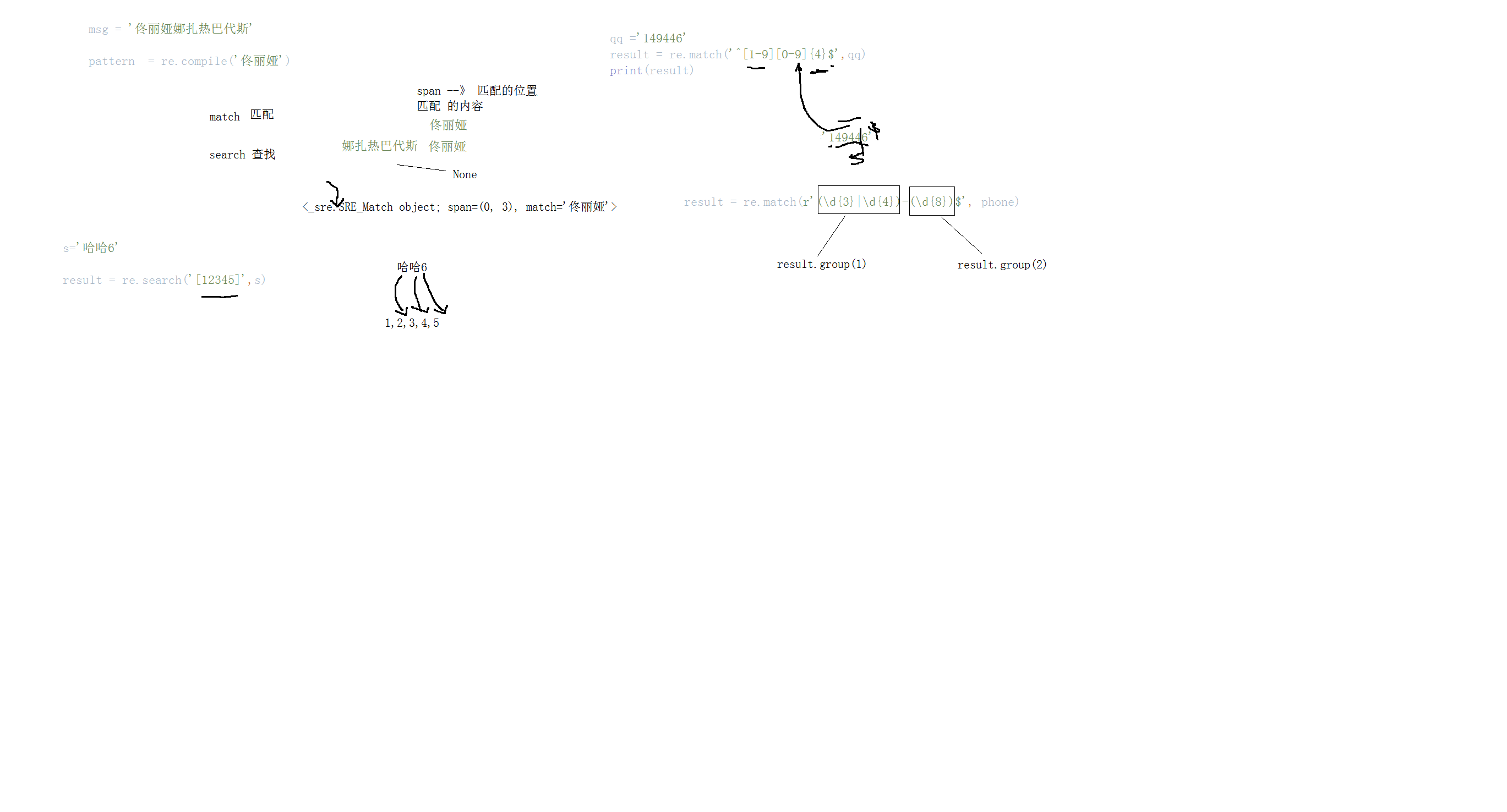# 正则表达式1896-刘同学

, ,

# 一、什么是正则表达式

1. 正则表达式的定义

10000 ~ 12数字组合
09876536
1. 长度
2. 是否是0开头

Regular Expression的“Regular”一般被译为“正则”、“正规”、“常规”。此处的“Regular”即是“规则”、“规律”的意思，Regular Expression即“描述某种规则的表达式”之意。

2. 正则表达式的作用和特点

1. 给定的字符串是否符合正则表达式的过滤逻辑（称作“匹配”）；
2. 可以通过正则表达式，从字符串中获取我们想要的特定部分。

1. 灵活性、逻辑性和功能性非常强；
2. 可以迅速地用极简单的方式达到字符串的复杂控制。
3. 对于刚接触的人来说，比较晦涩难懂。

<html><body><h1>hello world<h1></body></html>

s = "hello world"
start_index = s.find('')

python re模块：

/A：表示从字符串的开始处匹配
/Z：表示从字符串的结束处匹配，如果存在换行，只匹配到换行前的结束字符串。
/b：匹配一个单词边界，也就是指单词和空格间的位置。例如， 'py/b' 可以匹配"python" 中的 'py'，但不能匹配 "openpyxl" 中的 'py'。
/B：匹配非单词边界。 'py/b' 可以匹配"openpyxl" 中的 'py'，但不能匹配"python" 中的 'py'。
/d：匹配任意数字，等价于 [0-9]。  digit
/D：匹配任意非数字字符，等价于 [^/d]。not digit
/s：匹配任意空白字符，等价于 [/t/n/r/f]。 space
/S：匹配任意非空白字符，等价于 [^/s]。
/w：匹配任意字母数字及下划线，等价于[a-zA-Z0-9_]。
/W：匹配任意非字母数字及下划线，等价于[^/w]
//：匹配原义的反斜杠/。

‘.’用于匹配除换行符（/n）之外的所有字符。
‘^’用于匹配字符串的开始，即行首。
‘$’用于匹配字符串的末尾（末尾如果有换行符/n，就匹配/n前面的那个字符），即行尾。 定义正则验证次数： ‘*’用于将前面的模式匹配0次或多次（贪婪模式，即尽可能多的匹配） >=0 ‘+’用于将前面的模式匹配1次或多次（贪婪模式） >=1 ‘？’用于将前面的模式匹配0次或1次（贪婪模式） 0 ，1 '{m}' 用于验证将前面的模式匹配m次 '{m,}'用于验证将前面的模式匹配m次或者多次 >=m '{m,n}' 用于验证将前面的模式匹配大于等于m次并且小于等于n次 ‘*？，+？，？？’即上面三种特殊字符的非贪婪模式（尽可能少的匹配）。 ‘{m,n}’用于将前面的模式匹配m次到n次（贪婪模式），即最小匹配m次，最大匹配n次。 ‘{m,n}？’即上面‘{m,n}’的非贪婪版本。 ‘//’：'/'是转义字符，在特殊字符前面加上/，特殊字符就失去了其所代表的含义，比如/+就仅仅代表加号+本身。 ‘[]’用于标示一组字符，如果^是第一个字符，则标示的是一个补集。比如[0-9]表示所有的数字，[^0-9]表示除了数字外的字符。 ‘|’比如A|B用于匹配A或B。 ‘(...)’用于匹配括号中的模式，可以在字符串中检索或匹配我们所需要的内容。 Python里数量词默认是贪婪的（在少数语言里也可能是默认非贪婪），总是尝试匹配尽可能多的字符； 非贪婪则相反，总是尝试匹配尽可能少的字符。 在"*","?","+","{m,n}"后面加上？，使贪婪变成非贪婪。  # 二、正则表达式的简单应用 ## 1.简单使用 qq=input('输入qq号码') if len(qq)>=5 and qq !="0": print('合法的') else: print('不合法的') import re msg="佟丽娅娜扎热巴代斯" pattern=re.compile("佟丽娅") result=pattern.match(msg) #没有匹配 print(result)  ## 2. 正则表达式的方法 # 使用正则re模块方法, match() s="娜扎 佟丽娅 热巴代斯" result=re.match("佟丽娅",s) # 只要从开头进行匹配，如果匹配不成功则返回None print(result) result=re.search("佟丽娅",s) #search 进行正则字符串匹配方法 print(result) print(result.group()) #使用group提取到匹配的内容 print(result.span()) #返回位置 print(result.groups()) # a2b h6k #[] 表示的是一个范围 s="哈哈1a" result=re.search("[0-9][a-z]",s) print(result) msg="abcd7vikfd8hdf00" result= re.search("[a-z][0-9][a-z]",msg) #只要匹配到一个就不会再继续找 print(result.group()) result =re.findall("[a-z][0-9][a-z]",msg) # findall 匹配整个字符串 ，找到一个继续向下找，一直到结尾 print(result) #正则符号 # a7a a88a a7878a msg="a7asssda88asdffa7878a" #符号 + 出现一次或者多次, +号是放在匹配内容的后面 result= re.findall("[a-z][0-9]+[a-z]",msg) print(result) # qq 号认证 0-11 开头不能是零 qq="45743" re.match('^[1-9][0-9]{4,10}$',qq) #那整个正则表达式与qq的字符串进行匹配


## 3.正则表达式的简单应用

用户名可以是字母或者数字，不能是数字开头，用户名长度必须6位以上[0-9a-zA-Z]
result=re.match('[a-zA-Z]/w{5,}$',username) #/w：匹配任意字母数字及下划线，等价于[a-zA-Z0-9_]。 print(result) msg='aa.py ab.txt bb.py kk.png apyb.txt a*py' result=re.findall(r'/w*/.py/b',msg) print(result) ''' 总结: . 任意字符除(/n) ^ 开头$ 结尾
[] 范围 [abc]  [a-z] [a-z*&￥]

正则预定义:
/s 空白(空格)
/b 边界
/d 数字
/w  word [0-9a-zA-Z]

大写反面 /S 非空格  /D 非数字.......
'/w[0-9]'     ----> /w 和 [0-9]只能匹配一个字母

量词

*  >=0
+ >=1
? 0,1

手机号码的正则
re.match('1/d{9}$',phone) {m} : 固定m 位 {m,} >=m {m,n} phone >=m phone<=n  ## 4.正则表达的组合import re # 分组 # 匹配数字0-100数字 n='89' result=re.match('[1-9]?/d',n) print(result) ''' result=re.match('[1-9]+/d*',n) print(result) ''' # 改进版 result=re.match(r'[1-9]?/d?|$100$',n) print(result) # (word|word|word) 区别 [abc] 表示的是一个字母而不是一个单词 # 验证输入的邮箱 163 126 qq email='1570244983@qq.com' result=re.match(r'/w{2,20}@(162|126|qq)/.(com|cn)$',email)
print(result)

# 不是以4,7 结尾的电话号码
phone='17825352916'
result=re.match(r'1/d{9}[0-35-69]$',phone) print(result) #爬虫 phone = '010-12345678' result=re.match(r'(/d{3})|(/d{4})-(/d{8})$',phone)

# 分别提取
print(result.group())
#() 表示分组 group(1) 表示提取第一组的内容
print(result.group(1))
print(result.group(2))

#
msg='abc'
msg1='hello'

result=re.match(r'<[0-9a-zA-Z]+>(.+)',msg)
print(result)
print(result.group(1))

# number
result=re.match(r'<([0-9a-zA-Z]+)>(.+)$',msg1) print(result) print(result.group(1)) print(result.group(2)) # msg='abc' result=re.match(r'<([0-9a-zA-Z])>+<([0-9a-zA-Z]+)>(.+)$',msg)
print(result.group(1))
print(result.group(2))
print(result.group(3))

import re
# 起名的方式：  (?P<名字>正则)  (?P=名字)
msg = 'abc'
result=re.match(r'<(?P/w+)><(?P/w+)>(.+)',msg)
print(result)
print(result.group(1))
print(result.group(2))
print(result.group(3))
'''
分组：()   ---> result.group(1) 获取组中匹配内容
在分组的时候还可以结合 |
result = re.match(r'(/d{3}|/d{4})-(/d{8})$', phone) print(result) 不需要引用分组的内容： result = re.match(r'<[0-9a-zA-Z]+>(.+)', msg) print(result) print(result.group(1)) 引用分组匹配内容: 1.number /number 引用第number组的数据 msg = 'abc' result = re.match(r'<([0-9a-zA-Z]+)><([0-9a-zA-Z]+)>(.+)$',msg)
print(result)

2.?P<名字>
msg = 'abc'
result = re.match(r'<(?P/w+)><(?P/w+)>(.+)',msg)
print(result)
print(result.group(1))

re模块：
match    从开头匹配一次
search   只匹配一次
findall  查找所有
sub(正则表达式，'新内容'，string)   替换
split   result = re.split(r'[,:]','java:99,python:95')   在字符串中搜索如果遇到:或者,就分割
将分割的内容都保存到列表中了
'''

# 普通使用
result=re.sub(r'/d+','90','java:99,python:95')
print(result)

# 结合函数使用
def func(temp):
num=temp.group()
num1=int(num)+1
return  str(num1)


# 总结

re模块
import re

re.match(pattern,str)
re.search(pattern,str)
re.findall(pattern,str)
re.sub(pattern,'新的内容',str)  替换
re.split(pattern,str)  ---> []

. 任意字符
[] 范围
|  或者
()  一组

*  >=0
+  >=1
?  0,1
{m}  = m
{m,} >=m
{m,n}  >=m  <=n

预定义：
/s  space
/S  not space
/d  digit
/D  not digit
/w  word  [0-9a-zA-Z_]
/W  not word  [^0-9a-zA-Z_]
/b
/D

()   ---> group(1)

number
(/w+)(/d*)   ---> group(1)  group(2)
引用：
(/w+)(/d*)   /1  /2  表示引用前面的内容

name
(?P<name>/w+)   (?P=name)

Python里数量词默认是贪婪的（在少数语言里也可能是默认非贪婪），总是尝试匹配尽可能多的字符；

python
import re

# 默认是贪婪的
msg='abc123abc'
# 默认是贪婪1，如果想要将贪婪模式变成非贪婪的
result=re.match(r'abc(/d+)',msg)

print(result)

ath = ''

result = re.match(r', path)
# print(result.group(1))
image_path = result.group(1)
import requests

response = requests.get(image_path)

with open('aa.jpg', 'wb') as wstream:
wstream.write(response.content)

# 所有第三方库都有第三方的库


`

### 评论 抢沙发### 觉得文章有用就打赏一下文章作者

#### 支付宝扫一扫打赏#### 微信扫一扫打赏Vieu3.3主题Q Q 登 录